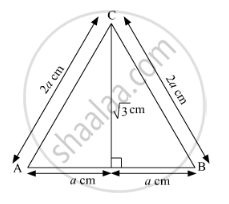Share

# Find the Length of the Side and Perimeter of an Equilateral Triangle Whose Height is √ 3 Cm. - Geometry

ConceptSimilarity in Right Angled Triangles

#### Question

Find the length of the side and perimeter of an equilateral triangle whose height is $\sqrt{3}$ cm.

#### SolutionSince, ABC is an equilateral triangle, CD is the perpendicular bisector of AB.
Now, According to Pythagoras theorem,
In ∆ACD

${AC}^2 = {AD}^2 + {CD}^2$
$\Rightarrow \left( 2a \right)^2 = a^2 + \left( \sqrt{3} \right)^2$
$\Rightarrow 4 a^2 - a^2 = 3$
$\Rightarrow 3 a^2 = 3$
$\Rightarrow a^2 = 1$
$\Rightarrow a = 1 cm$

Hence, the length of the side of an equilateral triangle is 2 cm.
Now,
Perimeter of the triangle = (2 + 2 + 2) cm
= 6 cm
Hence, perimeter of an equilateral triangle is 6 cm.

Is there an error in this question or solution?

#### APPEARS IN

Balbharati Solution for Balbharati Class 10 Mathematics 2 Geometry (2018 to Current)
Chapter 2: Pythagoras Theorem
Problem Set 2 | Q: 5 | Page no. 44

#### Video TutorialsVIEW ALL 

Solution Find the Length of the Side and Perimeter of an Equilateral Triangle Whose Height is √ 3 Cm. Concept: Similarity in Right Angled Triangles.
S